# SAT II Physics : Optics, Waves, and Quantum

## Example Questions

### Example Question #1 : Velocity, Frequency, And Wavelength

What is the wavelength of a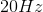wave if its velocity is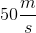?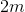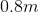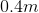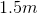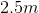Explanation:

The relationship between wavelength and velocity is given by the equation;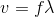The question gives us the velocity of the wave and its frequency. Using these values, we can solve for the wavelength.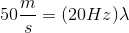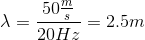### Example Question #1 : Waves

What best describes how a wave is affected when it travels from one medium to another medium?

The wave speed changed, but the frequency remains the same

Neither speed not frequency change

The wavelength does not change

Both speed and frequency change

The frequency changes, but the speed remains the same

The wave speed changed, but the frequency remains the same

Explanation:

It is a property of waves that the frequency remains constant and speed changes as it passes from one medium to another.

### Example Question #1 : Optics, Waves, And Quantum

A train blows its whistle as it approaches a station at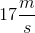. If the sound emitted by the whistle has a frequency of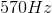, what is the frequency of the sound heard by the people at the station?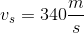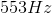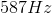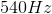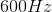Explanation:

Wave frequency is distorted when the observer or source is in motion based on the Doppler effect. The equation for the Doppler effect is:Terms in the numerator are summed if the observer moves toward the source. Terms in the denominator are summed if the source is moving away from the observer.

In this question, the train (source) is moving toward the observer. This means that the denominator terms will be subtracted. The velocity of the observer is zero, giving us our final equation: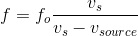Use the given values for the emitted frequency, velocity of the train, and speed of sound to solve this equation.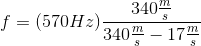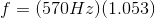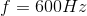### Example Question #1 : Doppler Effect

A dog is stationary in someone's front yard and barks at a frequency of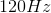. Which of the following could explain why an observer hears a frequency of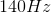?

Both the dog and the observer have the same acceleration

The observer is moving towards the dog

The observer is moving away from the dog

Both the dog and the observer have the same velocity

The observer is traveling in circular path with the dog at the center of the circle

The observer is moving towards the dog

Explanation:

The doppler effect states that the observed frequency depends on the frequency produced, and the relative velocities of the observer and sound source: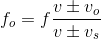To increase the observed frequency the sound source and the observer must be moving towards each other. Since the dog is stationary, therefore the observer must be moving towards the dog.

### Example Question #1 : Optics, Waves, And Quantum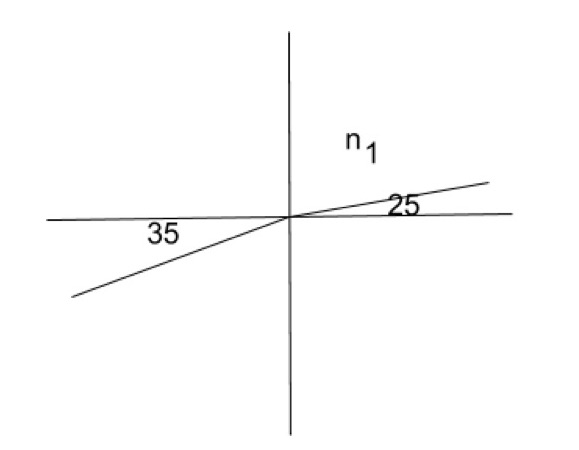Light passes from a medium with some index of refraction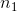at 25 degrees from perpendicular and exits into another medium with an index of refraction of 1.5 at an angle of 35 degrees. What is the value of?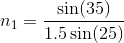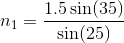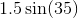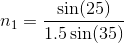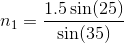Explanation:

Snell's law states: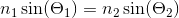If we substitute the values from the question we get: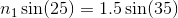Next rearrange to isolate### Example Question #1 : Optics, Waves, And Quantum

Which of the following scenarios would result in the polarization of light?

Light is reflected off of a glass window

Light passes through a vacuum

Light pass between two mediums with the same index of refraction

A light source travels at a high velocity

Light is absorbed by a black body

Light is reflected off of a glass window

Explanation:

Light is polarized when it is all oscillating in the same direction. This effect occurs when light is reflected. Polarization can occur through refraction, however if light is passing between mediums with the same index of refraction, the light will not refract.

### Example Question #1 : Optics

Of the following, which type of waves has the longest wavelength?

Microwaves

Gamma rays

Orange light

Green light

X-rays